# Resolution of singularities

(diff) ← Older revision | Latest revision (diff) | Newer revision → (diff)

desingularization

The replacement of a singular algebraic variety by a birationally isomorphic non-singular variety. More precisely, a resolution of singularities of an algebraic varietyover a ground fieldis a proper birational morphism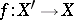such that the varietyis non-singular (smooth) (cf. Proper morphism; Birational morphism). A resolution of singularities of a scheme, of a complex-analytic space, etc. is defined analogously. The existence of a resolution of singularities enables one to reduce many questions to non-singular varieties, and in studying these one can use intersection theory and the apparatus of differential forms.

Usually a resolution of singularities is the result of successive application of monoidal transformations (cf. Monoidal transformation). It is known that if the centreof a monoidal transformation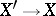is admissible (that is,is non-singular andis a normal flat variety along), then the numerical characteristics of the singularity of the variety (the multiplicity, the Hilbert function, etc.) are no worse than those of. The problem consists of choosing the centre of the blowing-up so that the singularities in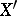really are improved.

In the case of curves the problem of resolution of singularities essentially reduces to normalization. In the two-dimensional case the situation is more complicated. The existence of a resolution of singularities for any variety over a fieldof characteristic zero has been proved. More precisely, for a reduced variety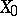there exists a finite sequence of admissible monoidal transformations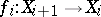,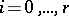, with centres, such that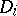is contained in the set of singular points ofandis a non-singular variety. An analogous result is true for complex-analytic spaces. In positive characteristic the existence of a resolution of singularities has been established (1983) for dimensions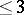.

The problem of resolution of singularities is closely connected with the problem of imbedded singularities, formulated as follows. Letbe imbedded in a non-singular algebraic variety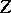. Does there exist a proper mapping, with non-singular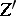, such that a)induces an isomorphism from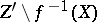onto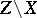; and b)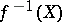is a divisor with normal crossings? (A divisor on a non-singular variety has normal crossings if it is given locally by an equation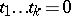, where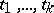are part of a regular system of parameters on.)

The problem of imbedded singularities is a particular case of the problem of trivialization of a sheaf of ideals. Letbe a non-singular variety, letbe a coherent sheaf of ideals onand letbe a non-singular closed subvariety. The weak pre-image of the idealunder a blowing-up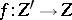with centre inis the sheaf of ideals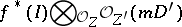on, whereandis the multiplicity of the idealat a regular point of. Trivialization of a sheaf of ideals consists of finding a sequence of blowing-ups with non-singular centres for which the weak pre-imagebecomes the structure sheaf. Letbe a non-singular variety over a field of characteristic zero, let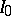be a coherent sheaf of ideals overand, in addition, let there be given a certain divisoronwith normal crossings. Then there exists a sequence of blowing-ups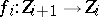,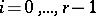, with non-singular centres, with the following properties: If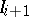is defined as the weak pre-image ofunder the blowing-upandis defined to be, then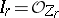, and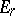has only normal crossings (Hironaka's theorem). In addition, one may assume thatlies in the set of points of maximal multiplicity of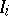and has normal crossings with. For positive characteristic an analogous result is known only when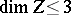.

Another problem of this type is the problem of eliminating points of indeterminacy of a rational transformation. Letbe a rational transformation of non-singular algebraic varieties. Does there exist a sequence of blowing-ups with non-singular centres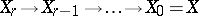such that the induced transformation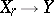is a morphism? This problem reduces to the problem of the existence of a trivialization of a sheaf of ideals, and the answer is affirmative ifor if.

How to Cite This Entry:
Resolution of singularities. Encyclopedia of Mathematics. URL: http://encyclopediaofmath.org/index.php?title=Resolution_of_singularities&oldid=12523
This article was adapted from an original article by V.I. Danilov (originator), which appeared in Encyclopedia of Mathematics - ISBN 1402006098. See original article